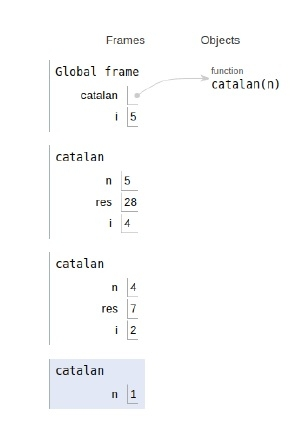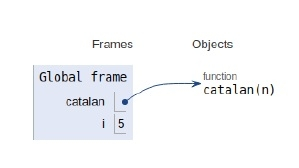# Python Program for nth Catalan Number

Catalan numbers are a sequence of natural numbers that are defined by the recursive formula −

$$C_{0}= 1\:and\:C_{n+1}=\displaystyle\sum\limits_{i=0}^n C_{i}C_{n-i} for \:n\geq0;$$

The first few Catalan numbers for n = 0, 1, 2, 3, … are 1, 1, 2, 5, 14, 42, 132,429,...................

Catalan numbers can be obtained both by recursion and dynamic programming. So let’s see their implementation.

## Approach 1: Recursion Method

#### Example

Live Demo

# A recursive solution
def catalan(n):
#negative value
if n <=1 :
return 1
# Catalan(n) = catalan(i)*catalan(n-i-1)
res = 0
for i in range(n):
res += catalan(i) * catalan(n-i-1)
return res
# main
for i in range(6):
print (catalan(i))

#### Output

1
1
2
5
14
42

The scope of all the variables and recursive calls are shown below.## Approach 2: Dynamic Programming Method

#### Example

Live Demo

# using dynamic programming
def catalan(n):
if (n == 0 or n == 1):
return 1
# divide table
catalan = [0 for i in range(n + 1)]
# Initialization
catalan = 1
catalan = 1
# recursion
for i in range(2, n + 1):
catalan[i] = 0
for j in range(i):
catalan[i] = catalan[i] + catalan[j] * catalan[i-j-1]
return catalan[n]
# main
for i in range (6):
print (catalan(i),end=" ")

#### Output

1
1
2
5
14
42

The scope of all the variables and recursive calls are shown below.